# Bridging the Gap- Modeling and Methods in Maths(Part 2)

there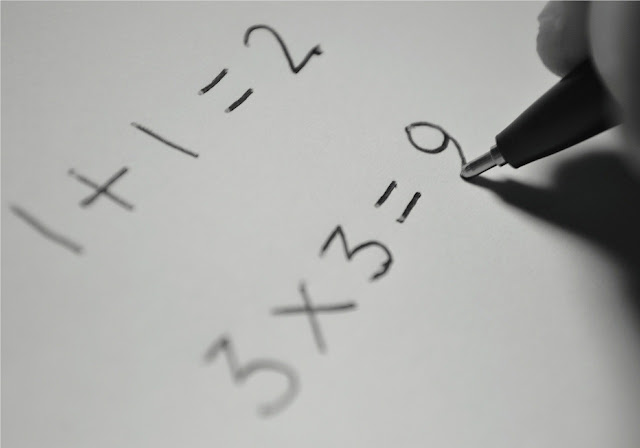Doing maths

Understanding Maths requires one to be able to analyse the question and choose the most appropriate method to solve the question. To be able to decide on which method  to use the child has to be able to  identify the crux of the  problem before he/she is able to slowly show the required workings to solve the problem.

## Using the Model Method

From Primary 1 students are taught to draw models to solve Long Answer Questions. This is the  method that is “promoted” as it is supposedly the easiest method to explain how you can derive the answer. ( And NO you are not allowed to even use Algebra to explain yourself)

Question 1

Sammy and Gerald had an equal number of stamps at first. Sammy then gave away 20 of this stickers to his friend Winston, and Sammy Gerald bought another 12 stamps. In the end, Gerald had thrice as many stickers as Sammy. Find the number of stamps Sammy had at first.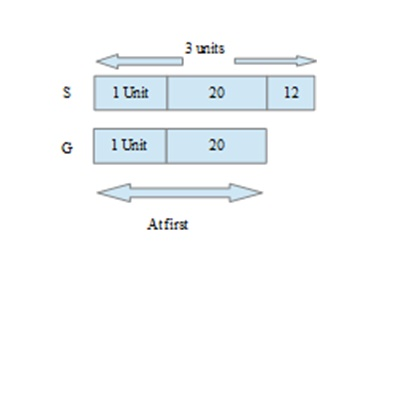Therefore 2 units 20 + 12

1 unit 32 2

1 unit → 16

Number of stamps Gerald had at first = 1 unit + 20

16 + 20

= 36

Gerald had 36 stamps at first.

If you notice the type of model drawn differs from the type of models drawn in lower primary.  It is more complex and you are comparing more then two different items involving multiple steps to solve the question.

## Looking at a Pattern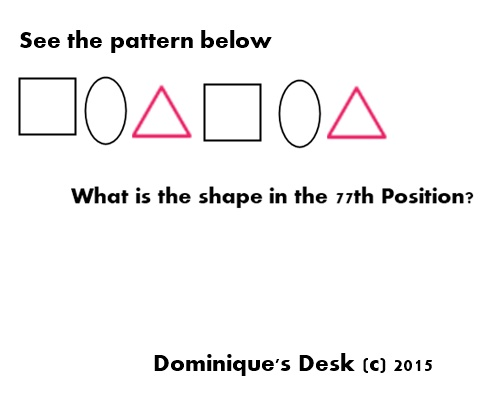Identifying the Pattern

How do you solve this problem?

Firstly you would need to identify the pattern.  Based on the diagram above you would notice that there are 3 different shapes – Square, Oval and Triangle.  The pattern repeats itself after the 3rd shape.

Step 1- Find out the number of “groups of 3” which are possible from 1- 77

77  3 = 25.66 ~ 25 times ( 25 R 2)

25x 3 = 75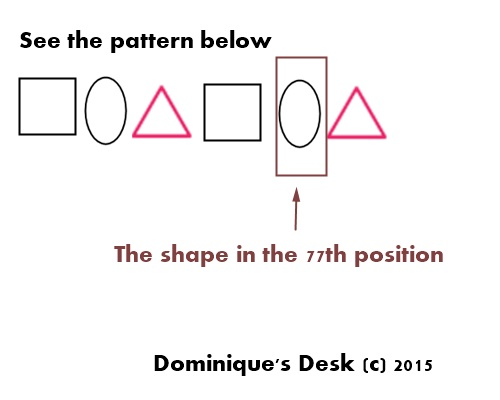It does take a lot of practice to be able to solve a mathematical question easily.   It’s fortunate that Maths is one of Monkey boy’s stronger subject and it doesn’t take him too much effort to understand the concepts which needs to be applied to the various questions.

## How to you reinforce the learning of Maths for your kids?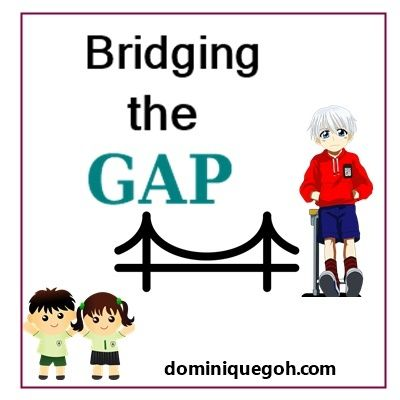Post in this series

9) Bridging the Gap- Upper and Lower Primary Science

10) Bridging the Gap-  Moving from Lower to Upper Primary

11) Bridging the Gap- Moving from Lower to Upper Primary ( Part 2)

# Bridging the Gap- Modeling and Methods in Maths(Part 1)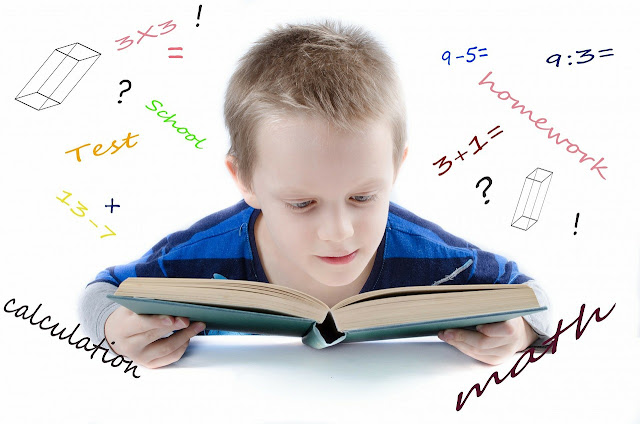Learning Maths

Maths is a subject which requires a lot of practice in order to be familiar with it. There are many different concepts which one need to master and formulas to memorise in order to complete the array of questions within the given time.

In the last post I gave an overview of the components tested in a Math’s paper.  In this post I will be showing how Long Answer Questions can be solved using different methods.

**These are actual questions which are taken from the homework worksheets that Monkey boy has brought  home and  I have worked through together with him.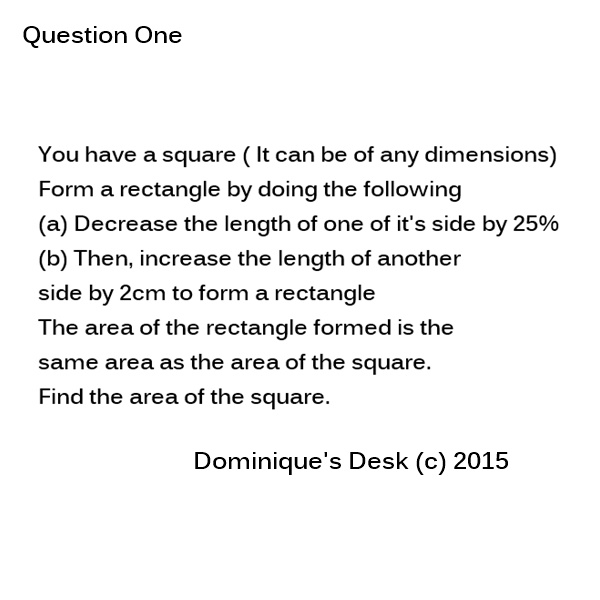Maths Question

My first inclination was to see if it was possible to draw a model to analyse the question.  After pondering on it for a while both of us found it more complicated to explain if we drew a model for it and eliminated it from the ” potential way to solve the question” list.

We decided to use the Guess/List and check method as there were two different unknowns which had to be identified.Square to rectangle

We worked through the possible  chance of  what the two different lengths of the rectangle could be given ( X and Y) given that the square was of a certain length for each side.

 Length of Side Area X (at 75%) Y at ( +2) Area of Rectangle 10 100cm² 10-2 = 8 10 + 2 = 12 8 x 12 = 94 cm² 8 64cm² 8- 2 = 6 8 +3 = 10 6 x 10= 60 cm² 6 36cm² 6-2.5=4.5 6=2= 8 4.5 x 8 = 36cm²

As shown above I assumed that each side of the square was 10cm so the area of the square would be 100 cm2.

Using the projections that ” one side had to be decreased by 25%”  the possibility of 1 side of the rectangle would  be 10-2 = 8 cm.

Following through on the part ” increase the length of the other side by 2cm” I added 2 to 10 to get 12cm.  However when you multiplied both sides to find the area of the rectangle I got 8 x 12 = 94 cm2.

This is not correct as there has been a change in the total area from 100cm2.    to  94 cm2.

I slowly moved down the list of possible numbers till I got to 6 cm as the length of the side of the square  and that the area of the square was equal to the area of the rectangle.

That was how we found the answer for this particular question.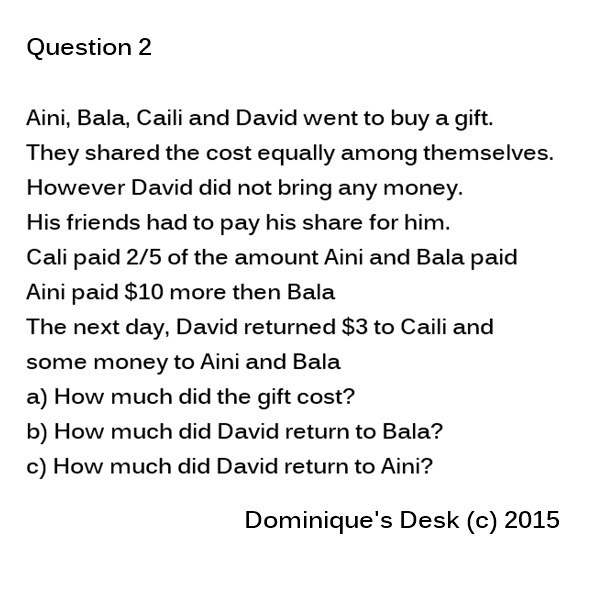If you are anything like me you too may draw a blank after reading through the whole question. I had to re-read through it a couple of times just to process what the questions was asking.  I found the question really complicated.

I seriously don’t remember having to attempt such complex questions when I was in Primary 5 back then. The difficultly level of questions attempted in Maths has certainly increased a lot!!

To solve this sum we had to use the Working backward method.

We start off from the sentence

” David paid \$3 back to Cali”

If we divide the amount David should have paid in 2 parts

The amount Cali paid : The amount  Aini and Bala paid = 2u: 5u

Therefore if all the kids paid  and equal proportion of the present  each

2 u (x4) : 5 u (x4)

8 u : 20 u

Cost of the present = 28u

Each child would have to pay 7 u. However Calia paid  1u more as she paid part of David share so

8u- 7u = 1 u

1 u = \$3

28 u = \$3 x 28

= \$84

a) The price of the present is \$84

The amount of money Aini and Bala paid in total = 20 u (\$60)

Based on the fact that Aini paid \$10 more then Bala we find the amount the Aini paid by doing the following.  (60 + 10) / 2 = \$35

Bala pays \$10 lesser

\$35 – \$10 = \$25

(Checking – \$25 + \$35 = \$60)

Since each person originally needed to pay 7 u = \$21

David had to return \$25-\$21 = \$4 to Bala  and \$35-\$21 = \$14 to Aini

b) David returns \$4 to Bala

c) David returns \$14 to Aini.

In the next post I will go through the remaining two methods- Modelling and Looking at a pattern to show how they can be applied in problem solving.Post in this series

9) Bridging the Gap- Upper and Lower Primary Science

10) Bridging the Gap-  Moving from Lower to Upper Primary

11) Bridging the Gap- Moving from Lower to Upper Primary ( Part 2)